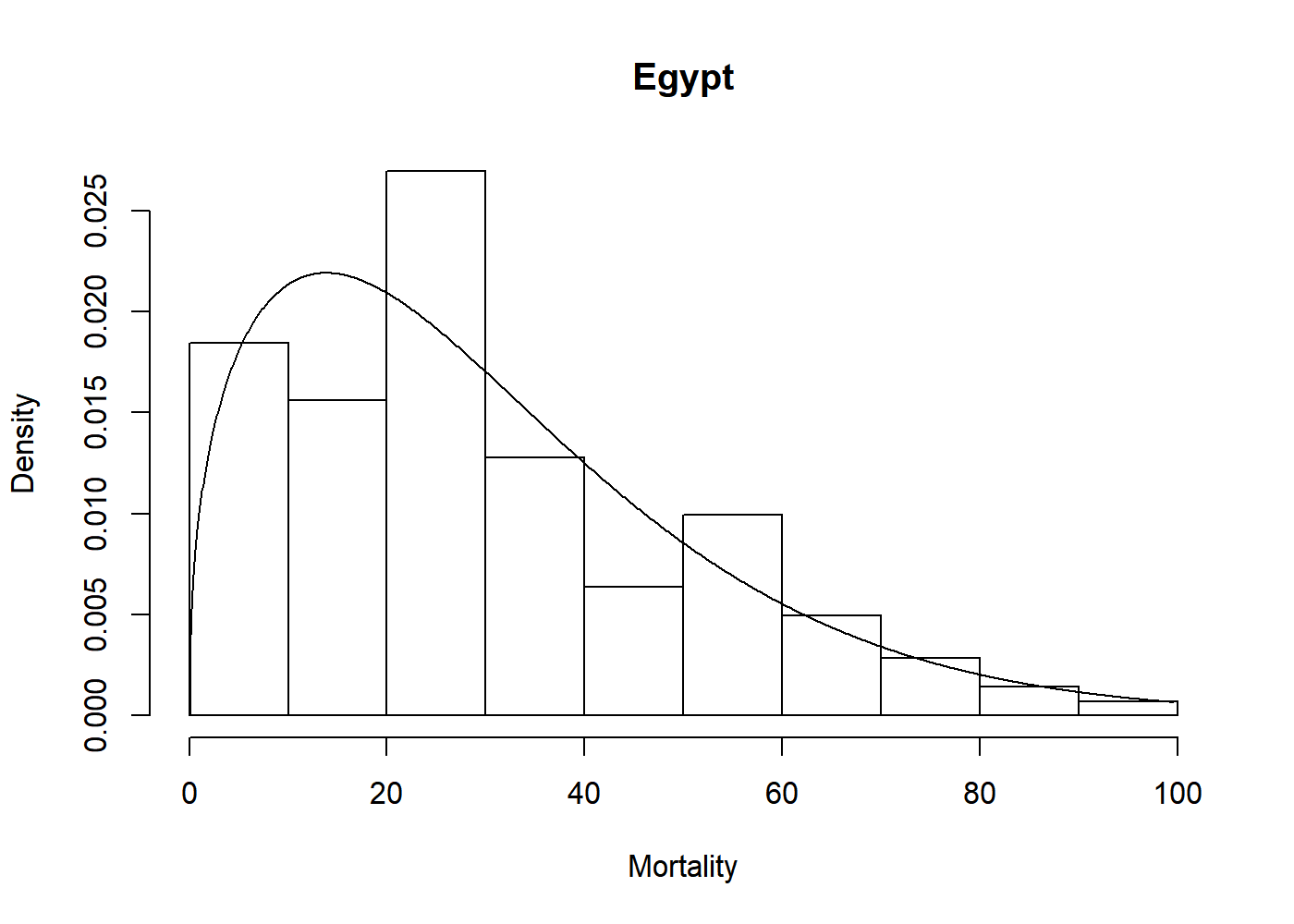Overview

An R-package for fast, easy, and reliable maximum likelihood estimation for a selection of parametric univariate densities. In addition to basic estimation capabilities, this package support visualization through plot and qqmlplot, model selection by AIC and BIC, confidence sets through the parametric bootstrap with bootstrapml, and convenience functions such as the density, distribution function, quantile function, and random sampling at the estimated distribution parameters.

Installation

Use the following command from inside R:

Usage

The core of univariateML are the ml*** functions, where *** is a distribution suffix such as norm, gamma, or weibull.

Now we can visually assess the fit of the Weibull model to the egypt data with a plot.

hist(egypt$age, freq = FALSE, xlab = "Mortality", main = "Egypt") lines(mlweibull(egypt$age))Implementations

Analytic formulae for the maximum likelihood estimates are used whenever they exist. Most ml*** functions without analytic solutions have a custom made Newton-Raphson solver. These can be much faster than a naïve solution using nlm or optim. For example, mlbeta has a large speedup over the naïve solution using nlm.

The maximum likelihood estimators in this package have all been subject to testing, see the tests folder for details.

Documentation

For an overview of the package and its features see the overview vignette. For a list of implemented densities see the start of the details vignette. For an illustration of how this package can make an otherwise long and laborious process much simpler, see the copula vignette.